# Burn down chart per projection needed per day and real expenses

Hello to all i’m trying to create a burndown chart per projection where

projection = total planned hours in the sprint / productivity needed per day

this same formula above in dividing all working days as in the print of excel exemplo

and the real expenses that is

real expenses = total planned hours in the sprint / (real )real time spent

this same formula above in dividing all working days as in the print of excel exemplo

A print of both formulas in excel and a chart for ilustrate and a file to dowload to understand

i would like to create this in eazy bi but how can i do this ? i have no idea how to make this, how to create a repetitive loop to dividing total hours over real per day in sprint

2 Likes

Hi,

You can use the visiblerowset to find the first item.

Try creating new calculated measure using this formula:

``````(VisibleRowsSet().Item(0).Item(0),
[Measures].[Total planned hours in the sprint that I will spend]
)
-
Sum(
{
Filter(
[Time].CurrentHierarchyMember.level.Members,
Rank(
[Time].CurrentHierarchyMember,
VisibleRowsSet()
) = 1
).item(0):
[Time].CurrentHierarchyMember},
[Measures].[Real]
)

``````

Martins / eazyBI

1 Like

can you show me measure of burndown values and start value ?

@Lucas_Silva_Dantas_A

“start value” is just the simple integer “48”

“Burnndown values” is the formula from above:

``````(VisibleRowsSet().Item(0).Item(0),
[Measures].[Total planned hours in the sprint that I will spend]
)
-
Sum(
{
Filter(
[Time].CurrentHierarchyMember.level.Members,
Rank(
[Time].CurrentHierarchyMember,
VisibleRowsSet()
) = 1
).item(0):
[Time].CurrentHierarchyMember},
[Measures].[Real]
)
``````

Martins / eazyBI

1 Like

isn’t worked for me, the values are returning negative or with no sense print of values that are returning

Start value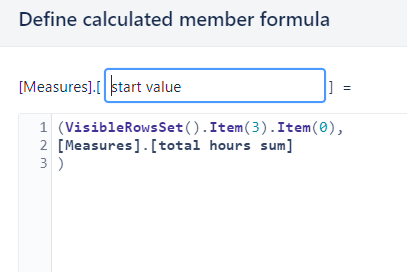Total planned hours in the sprint that I will spend

real 2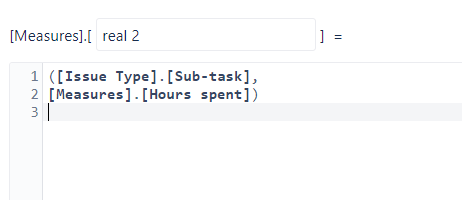with the values selected would return the values like this

and this is what happening when i change the measures puting start value as first field and planned in second, almost got correct

** ------ ------ ------ ------The correct- ------ ------ ------**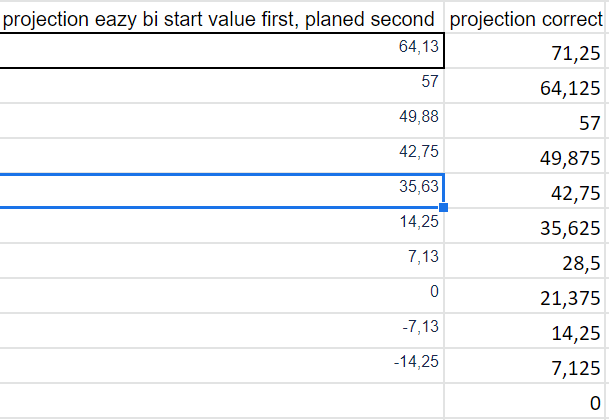@martins.vanags this is possibly happening because is considering weekend, there is a way to desconsidere the weekend ?

@Lucas_Silva_Dantas_A

Try to add “Week Day” dimension as a page filter and select all dates but Saturday and Sunday (using multi-value selector)

Martins

i solved this problem, following these steps

1. i added Sprint in pages and Time dimension in rows the time with hierarchy level as day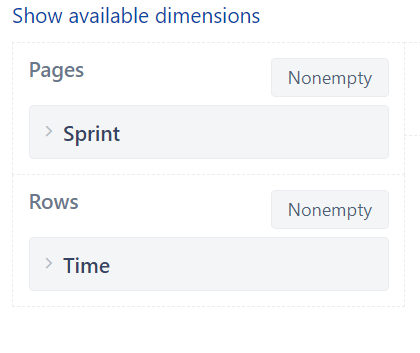2. i created a measure called working days to remove saturday and sundays added the measure and filtered >0 i made the same thing with the time within sprint that already comes in eazybi by default and i removed both coluns

``````[Measures].[ Working days ]  =

DateDiffWorkDays(
[Time].CurrentHierarchyMember.StartDate,
[Time].CurrentHierarchyMember.NextStartDate
)
``````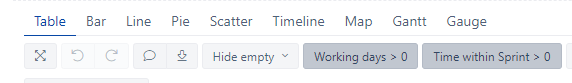3. to create the projection without real expense i created three measure the first measure i called by total hours sum v3
the code is below

``````[Measures].[ total hours sum v3]  =

Sum(
{PreviousPeriods([Time].CurrentHierarchyMember),
[Time].CurrentHierarchyMember}
,

[Measures].[Original estimated hours]
)

``````

Then i created the second measure called start value v3, for this i used de following code , i used the item 9 because my squads usually add more hours during the sprint and this value change, só he takes the final value

``````[Measures].[start value v3]  =

(VisibleRowsSet().Item(9).Item(0),
[Measures].[total hours sum v3]
)
``````

And finally i created the measure projeção that means projection in english, using the other measures that i created following the code bellow

``````-- annotations.group = Time tracking
CASE WHEN
DateBetween([Time].CurrentHierarchyMember.StartDate,
DateWithoutTime([Sprint].CurrentMember.get('Start date')),
[Sprint].CurrentMember.get('End date')
)
OR
DateInPeriod(
[Sprint].CurrentMember.get('Start date'),
[Time].CurrentHierarchyMember
)
THEN
([Measures].[start value v3],
[Time].CurrentHierarchy.DefaultMember) *
(
DateDiffWorkdays(
DateWithoutTime([Sprint].CurrentMember.get('Start date')),
DateWithoutTime([Sprint].CurrentMember.get('End date'))
) -
DateDiffWorkdays(
DateWithoutTime([Sprint].CurrentMember.get('Start date')),
[Time].CurrentHierarchyMember.StartDate
)
) /
DateDiffWorkdays(
DateWithoutTime([Sprint].CurrentMember.get('Start date')),
DateWithoutTime([Sprint].CurrentMember.get('End date'))
)
END
``````

5. to create the real expenses i too used star value v3 measure and but i too used a measure called Horas gasts that means hours spent the horas gastas measure code bellow

``````[Measures].[ Horas gastas]  =

[Measures].[Hours spent])
``````

and then i finally create the real expense(gastos reais) measure following the code below

``````(VisibleRowsSet().Item(0).Item(0),
[Measures].[start value v3]
)
-
Sum(
{
Filter(
[Time].CurrentHierarchyMember.level.Members,
Rank(
[Time].CurrentHierarchyMember,
VisibleRowsSet()
) = 1
).item(0):
[Time].CurrentHierarchyMember},
[Measures].[Horas gastas]
)
``````

i noticed a differençe of 1,3 between it’s happening is that because i started the sprint near the end of the day but the value is correct.

final result

3 Likes